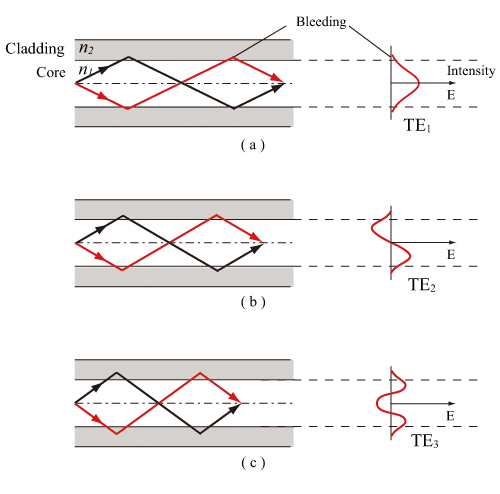The fundamental mode corresponds to the maximum incident angle to optical fiber (the mode closest to the fiber axis). The higher-order mode corresponds to the smaller incident angle compared with the fundamental mode. Provided that each mode is denoted as Nth-order mode, N is mode number. N represents the number of nodes in electric field distribution in the transverse direction. For the fundamental mode, N equals to 0. Figure shows the field distributions of transverse electric (TE) mode corresponding to each mode number. The larger the mode number is, the larger the light leaking from the core to the clad is. Optical fiber usable for propagation of a number of modes is multimode optical fiber (MMF), optical fiber only for the fundamental mode propagation is singlemode optical fiber (SMF). The difference between SMF and MMF is yielded mainly from the core diameter difference. The relative refractive index difference of typical SMF is ca. 0.3 %, and the typical core diameter is 2~10 µm. The core diameter of MMF is in the range 7 µm~3 mm, and typically is 50, 62.5, 100, or 200 µm. Particularly, in MMF used for optical communication, the core diameter is mostly either 50 or 62.5 µm.Fig. Mode number and an electric field distribution of mode. (a) N=0, (b) N=1, (c) N=2